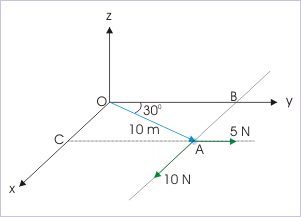# 18.1 Torque  (Page 2/3)

 Page 2 / 3

By the definition of vector product, the torque is perpendicular to the plane formed by the position vector and force. Besides, it is also perpendicular to each of the two vectors individually. However, the vector relation by itself does not tell which side of the plane formed by operands is the direction of torque. In order to decide the orientation of the torque, we employ right hand vector product rule.

For this, we need to shift one of the operand vectors such that their tails meet at a point. It is convenient to shift the force vector, because application of right hand vector multiplication rule at the point (origin of the coordinate system) gives us the sense of angular direction of the torque.

In the figure, we shift the second vector ( F ) so that tails of two operand vectors meet at the point (origin) about which torque is calculated. The two operand vectors define a plane ("xy" - plane in the figure). The torque ( τ ) is, then, acting along a line perpendicular to this plane and passing through the meeting point (origin).

The orientation of the torque vector in either of two direction is determined by applying right hand rule. For this, we sweep the closed fingers of the right hand from position vector (first vector) to force vector (second vector). The outstretched thumb, then, indicates the orientation of torque. In the case shown, the direction of torque is positive z-direction.

While interpreting the vector product, we must be careful about the sequence of operand. The vector product " $\mathbf{F}\mathbf{x}\mathbf{r}$ " is negative or opposite in sign to that of " $\mathbf{r}\mathbf{x}\mathbf{F}$ ".

## Illustration

The problem involving torque is about evaluating the vector product. We have the options of using any of the three methods described above for calculating the magnitude of torque. In this section, we shall work with two important aspects of calculating torque :

• More than one force operating simultaneously on the particle
• Relative orientation of the plane of operand vectors and planes of coordinate system

## More than one force operating simultaneously on the particle

Here, we have two choices. Either, we evaluate torques for each force and then find the resultant torque or we evaluate the resultant (net) force first and then find the torque. The choice depends on the situation in hand.

In particular, if we find that the resulting torques are along the same direction (say one of the coordinate direction), then it is always better to calculate torque individually. This is because torques along a direction can be dealt as scalars with appropriate sign. The resultant torque is simply the algebraic sum. Example below highlights the this aspect.

The bottom line in making choice is that we should keep non-linear vector summation - whether that of force or torque - to a minimum. Otherwise, analytical technique for vector addition will be need to be employed.

Problem : At an instant, a particle in xy plane is acted by two forces 5 N and 10 N in xy plane as shown in the figure. Find the net torque on the particle at that instant.Two forces are acting simultaneously on the particle.

Solution : An inspection of the figure reveals that forces are parallel to axes and perpendicular linear distances of the lines of forces can be known. In this situation, calculation of the magnitude of torque suits to the form of expression that uses moment arm. Here, moment arms for two forces are :

For 5 N force, the moment arm "AB" is :

$\begin{array}{l}\mathrm{AB}=\mathrm{OA}\mathrm{sin}{30}^{0}=10x0.5=5\phantom{\rule{2pt}{0ex}}m\end{array}$

For 10 N force, the moment arm "AC" is :

$\begin{array}{l}\mathrm{AC}=\mathrm{OA}\mathrm{cos}{30}^{0}=10x0.866=8.66\phantom{\rule{2pt}{0ex}}m\end{array}$

The torques due to 5 N is :

$\begin{array}{l}⇒{\tau }_{1}=5x5=25\phantom{\rule{4pt}{0ex}}Nm\phantom{\rule{4pt}{0ex}}\text{(positive being anti-clockwise)}\end{array}$

The torques due to 10 N is :

$\begin{array}{l}⇒{\tau }_{2}=-8.66x10=-86.6\phantom{\rule{4pt}{0ex}}Nm\phantom{\rule{4pt}{0ex}}\text{(negative being clockwise)}\end{array}$

Net torque is :

$\begin{array}{l}⇒{\tau }_{\mathrm{net}}={\tau }_{1}+{\tau }_{2}=25-86.6=-61.6\phantom{\rule{4pt}{0ex}}Nm\phantom{\rule{4pt}{0ex}}\text{(negative being clockwise)}\end{array}$

We can also first calculate the resultant force and then apply the definition of torque to obtain net torque. But, then this would involve non-linear vector addition.

#### Questions & Answers

A stone propelled from a catapult with a speed of 50ms-1 attains a height of 100m. Calculate the time of flight, calculate the angle of projection, calculate the range attained
58asagravitasnal firce
Amar
water boil at 100 and why
what is upper limit of speed
what temperature is 0 k
Riya
0k is the lower limit of the themordynamic scale which is equalt to -273 In celcius scale
Mustapha
How MKS system is the subset of SI system?
which colour has the shortest wavelength in the white light spectrum
how do we add
if x=a-b, a=5.8cm b=3.22 cm find percentage error in x
x=5.8-3.22 x=2.58
what is the definition of resolution of forces
what is energy?
Ability of doing work is called energy energy neither be create nor destryoed but change in one form to an other form
Abdul
motion
Mustapha
highlights of atomic physics
Benjamin
can anyone tell who founded equations of motion !?
n=a+b/T² find the linear express
أوك
عباس
Quiklyyy
Moment of inertia of a bar in terms of perpendicular axis theorem
How should i know when to add/subtract the velocities and when to use the Pythagoras theorem?
Centre of mass of two uniform rods of same length but made of different materials and kept at L-shape meeting point is origin of coordinateByBy Mistry BhaveshBy